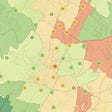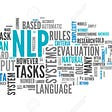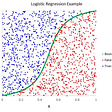# One parameter

• Let’s suppose true phenomenon is
`y <- 6x`

• but we don’t know the exact relationship so we assume

`y <- ax`

• using sum of squared errors as the loss function

`yhat <- ax1 residual <- yhat — y residual <- ax-y  residual² <- (ax — y)²• let residual² = loss function()• so lossfunction() <- (ax — y)²`
• Only thing we can vary the residuals with is by changing a. x and y are constants given by our dataset.
• Consider the differential below
`d lossfunction() — — — — — — — — <- 2*(ax-y) d a`

Lets see how change in a changes residuals

`library(ggplot2) library(dplyr) library(tidyr) a <- c(1,2,3,4,5,6,7,8,9,10,11)  x <- c(8,9,10,11,12,13) y <- 6*x + rnorm(length(x) , sd = 1.5) # random noise  residuals <- sapply(a, function(a) sum((a*x — y)²) )  ggplot(data = data.frame(coff = a , res = residuals), aes(x = coff , y = res)) + geom_point() + geom_smooth()`
• We expected a parabola because
`lossfunction() <- \$_ (ax — y)²`

• We see that the minimum residuals are achieved when coff = 6 which is our true parameter.

• what if we had 2 params

# Two Parameters

• Suppose our true relationship is

`y = 7*x1 + 2*x2`

• about which we don’t know so we assume

`y = a*x1 + b*x2`

• we want to find best a and b that describe my data/fit my data well.

• we again use our least square loss function.

`residual = (yhat — y) = (ax1 + bx2 — y)  residual² = (ax1 + bx2 — y)²`

• what we can change here are both a and b.

`so d lossfunction() — — — — — — — = 2x1(ax1 + bx2 — y) d aso d lossfunction() — — — — — — = 2x2(ax1 + bx2 — y) d b`

But now its difficult for us to visualize since there are 3 dimensions 1. Total Loss 2. a 3. b

`a <- c(4,5,6,7,8,9,10) b <- c(-1,0,1,2,3,4,5)  x1 <- c(8,9,10,11,12,13) x2 <- c(100,120,130,140,80,70)   #since its linear combination we would want to scale all the independent variables  work <- function(x1 , x2, a,b){  x1 <- scale(x1) x2 <- scale(x2)  # True # y = 7*x1 + 2*x2  y <- 7*x1 + 2*x2 +rnorm(length(x) , sd = 1.5) # random noise  ecomb <- expand.grid(a,b) %>% as.data.frame() colnames(ecomb) <- c(“a” , “b”)  ecomb\$residuals <- sapply(a, function(a) sapply(b , function(b) sum((a*x1 + b*x2 — y)²))) %>%  matrix(nrow = length(a)*length(b))  head(ecomb %>% filter(a == 7 | b == 2))  gathered <- ecomb %>% gather(key=”coefname” , value=”coefvalue” , -residuals)  ggplot(data =gathered , aes(x = coefvalue , y = residuals) ) + geom_point(width = 0.1) +  geom_smooth(color = ‘green’) + geom_hline(yintercept = 0 , color = ‘blue’) + facet_grid(~coefname) + geom_vline(data = data.frame(coefname=c(“a” , “b”) , z=c(7.0,2.0)) , aes(xintercept = z) , color = ‘yellow’)  }  work(x1 ,x2, a,b)`

We get slight errors in our a and b estimate but thats due to small sample size. If we increase the sample size to be 100 instead of 7

`## Same coef values as above a <- c(4,5,6,7,8,9,10) b <- c(-1,0,1,2,3,4,5)   ### Increased Sample Size to 100 x1 <- rnorm(100 , 10 , 5) x2 <- rnorm(100 , 100 , 10)   work(x1 ,x2, a,b)`

• Now the idea is to use a loss function and an algorithm that would find the minima of our loss function. One such algorith is gradient descent.

• The algorithm would travel the green line in our above examples and find the minima and therefore the corresponding values of a and b which are minimizing the loss function (in our case above — Sum of squared errors)

• No matter how many parameters concept remains the same — to find the minima of the loss function

• Lets return the simple 1 parameter example

`a <- c(1,2,3,4,5,6,7,8,9,10,11)  x <- rnorm(100) y <- 6*x + rnorm(length(x) , sd = 1.5) # random noise  residFunc <- function(a) sum((a*x — y)²)  residuals <- sapply(a, residFunc)  slope = function(a){ sum(2*x*(a*x — y)) }   learningRate <- 0.001 startingA <- 11   gradiantDescent <- function(startingA, learningRate , iter = 10){ aestimate <- startingA estimates <- c() stepStop <- 0.1  for( i in seq(1,iter,1) ){  step <- slope(aestimate)*learningRate if(abs(step) < stepStop) break newAEstimate <- aestimate — step aestimate <- newAEstimate print(aestimate) estimates <- c(estimates , aestimate) } estimates }  estimates <- gradiantDescent(startingA , learningRate , 20) 10.13894  9.421883  8.824738  8.327455  7.913334  7.568467  7.281272  7.042106  6.842936  6.677074  6.538949  6.423924`
`ggplot(data = data.frame(coff = a , res = residuals), aes(x = coff , y = res)) + geom_point() + geom_smooth() + geom_point(data = data.frame(x = estimates , y = sapply(estimates, residFunc )) , aes(x=x, y=y) , color = ‘red’ ,size=5)ggplot(data = data.frame(x = estimates ), aes(y = x , x = seq(1:length(x)))) + geom_point() + geom_smooth()`
• What if we change our starting a estimate or our learning rate
`startingA = 100 learningRate = 0.001 iter = 100  estimates <- gradiantDescent(startingA , learningRate , iter) 84.25529  71.14359  60.2246  51.13161  43.55926  37.25325  32.00181  27.62857  23.98669  20.95384  18.42818  16.32489  14.57334  13.11471  11.90001  10.88844  10.04604  9.344515  8.760308  8.2738  7.868652  7.531257  7.250285  7.016301  6.821447  6.659178  6.524046  6.411513ggplot(data = data.frame(x = estimates ), aes(y = x , x = seq(1:length(x)))) + geom_point() + geom_smooth() + geom_hline(yintercept = 6 , color = ‘red’)`

We can see that it converges to the truth of 6

• Lets change the learning rate
`startingA = 100 learningRate = 0.002 iter = 100  estimates <- gradiantDescent(startingA , learningRate , iter) 68.51057  47.55323  33.60537  24.32257  18.14453  14.03283  11.29634  9.475114  8.26302  7.456329  6.919446  6.562132  6.324327  6.166058  6.060725ggplot(data = data.frame(x = estimates ), aes(y = x , x = seq(1:length(x)))) + geom_point() + geom_smooth() + geom_hline(yintercept = 6 , color = ‘red’)`
• We see that the algorithm converges much more quickly when we increase the learning rate

• But what if we increase it too much ?

`startingA = 100 learningRate = 0.005 iter = 100  estimates <- gradiantDescent(startingA , learningRate , iter) 21.27643  8.378402  6.265195  5.918968ggplot(data = data.frame(x = estimates ), aes(y = x , x = seq(1:length(x)))) + geom_point() + geom_smooth() + geom_hline(yintercept = 6 , color = ‘red’)`
• Converges at an even faster rate
`startingA = 100 learningRate = 0.1 iter = 100  estimates <- gradiantDescent(startingA , learningRate , iter) -1474.471  23281.27  -365958.5  5754140  -90473445  1422533037  -22366785121  351677652498  -5.529501e+12  8.694151e+13  -1.367e+15  2.149362e+16  -3.379487e+17  5.313639e+18  -8.354745e+19  1.313634e+21  -2.065454e+22  3.247557e+23  -5.106203e+24  8.028589e+25  -1.262352e+27  1.984822e+28  -3.120778e+29  4.906865e+30  -7.715166e+31  1.213072e+33  -1.907338e+34  2.998948e+35  -4.715308e+36  7.413978e+37  -1.165715e+39  1.832879e+40  -2.881874e+41  4.53123e+42  -7.124548e+43  1.120208e+45  -1.761326e+46  2.76937e+47  -4.354338e+48  6.846417e+49  -1.076477e+51  1.692567e+52  -2.661258e+53  4.184352e+54  -6.579144e+55  1.034453e+57  -1.626492e+58  2.557367e+59  -4.021001e+60  6.322305e+61  -9.940692e+62  1.562996e+64  -2.457531e+65  3.864028e+66  -6.075492e+67  9.552624e+68  -1.501979e+70  2.361593e+71  -3.713182e+72  5.838315e+73  -9.179704e+74  1.443344e+76  -2.2694e+77  3.568226e+78  -5.610396e+79  8.821343e+80  -1.386998e+82  2.180807e+83  -3.428928e+84  5.391375e+85  -8.476972e+86  1.332852e+88  -2.095671e+89  3.295068e+90  -5.180904e+91  8.146045e+92  -1.28082e+94  2.01386e+95  -3.166434e+96  4.97865e+97  -7.828036e+98  1.230819e+100  -1.935242e+101  3.042821e+102  -4.784292e+103  7.522442e+104  -1.182769e+106  1.859693e+107  -2.924034e+108  4.597521e+109  -7.228778e+110  1.136596e+112  -1.787094e+113  2.809885e+114  -4.418041e+115  6.946578e+116  -1.092225e+118  1.717328e+119  -2.700191e+120  4.245567e+121ggplot(data = data.frame(x = estimates ), aes(y = x , x = seq(1:length(x)))) + geom_point() + geom_smooth() + geom_hline(yintercept = 6 , color = ‘red’)`

estimates

` -1.474471e+03 2.328127e+04 -3.659585e+05 5.754140e+06 -9.047344e+07  1.422533e+09 -2.236679e+10 3.516777e+11 -5.529501e+12 8.694151e+13  -1.367000e+15 2.149362e+16 -3.379487e+17 5.313639e+18 -8.354745e+19  1.313634e+21 -2.065454e+22 3.247557e+23 -5.106203e+24 8.028589e+25  -1.262352e+27 1.984822e+28 -3.120778e+29 4.906865e+30 -7.715166e+31  1.213072e+33 -1.907338e+34 2.998948e+35 -4.715308e+36 7.413978e+37  -1.165715e+39 1.832879e+40 -2.881874e+41 4.531230e+42 -7.124548e+43  1.120208e+45 -1.761326e+46 2.769370e+47 -4.354338e+48 6.846417e+49  -1.076477e+51 1.692567e+52 -2.661258e+53 4.184352e+54 -6.579144e+55  1.034453e+57 -1.626492e+58 2.557367e+59 -4.021001e+60 6.322305e+61  -9.940692e+62 1.562996e+64 -2.457531e+65 3.864028e+66 -6.075492e+67  9.552624e+68 -1.501979e+70 2.361593e+71 -3.713182e+72 5.838315e+73  -9.179704e+74 1.443344e+76 -2.269400e+77 3.568226e+78 -5.610396e+79  8.821343e+80 -1.386998e+82 2.180807e+83 -3.428928e+84 5.391375e+85  -8.476972e+86 1.332852e+88 -2.095671e+89 3.295068e+90 -5.180904e+91  8.146045e+92 -1.280820e+94 2.013860e+95 -3.166434e+96 4.978650e+97  -7.828036e+98 1.230819e+100 -1.935242e+101 3.042821e+102 -4.784292e+103  7.522442e+104 -1.182769e+106 1.859693e+107 -2.924034e+108 4.597521e+109  -7.228778e+110 1.136596e+112 -1.787094e+113 2.809885e+114 -4.418041e+115  6.946578e+116 -1.092225e+118 1.717328e+119 -2.700191e+120 4.245567e+121`

• Now what we see is that it fails to converge. Begins to diverge and then never looks back.

--

--

--

School of Data Science @ University of North Carolina — Charlotte

Love podcasts or audiobooks? Learn on the go with our new app.

## Metric Learning with Catalyst## Training a CNN to detect Face Masks## Music Through Machine Learning## TF-IDF vs. Word2Vec Vectorization Techniques for Twitter Sentiment Analysis## Teaching a Xenophobic AI Health Inspector, and the Ethics of Machine Learning## How to Succeed in Multilingual Sentiment Analysis without Transformers## Convolutional Neural Networks## Convert StyleGAN2 TensorFlow weights to Pytorch## Abhijeet Pokhriyal

School of Data Science @ University of North Carolina — Charlotte

## Logistic Regression